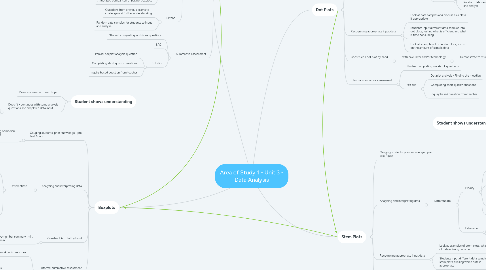# Area of Study 1 - Unit 3 - Data Analysisgeorgia byron
Get Started. It's FreeArea of Study 1 - Unit 3 - Data Analysis## 1. Dot Plots

### 1.2. Analysing and interpreting data

1.2.1. Differentiate

1.2.1.1. Modify

1.2.1.1.1. Demonstrate with examples of the board

1.2.1.1.2. Small focus group with teacher to work on questions together

1.2.1.1.3. Template or checklist for students to follow, what should be included when students respond to describing and comparing data

1.2.1.1.4. Annotated dot plot example

1.2.1.2. Extension

1.2.1.2.1. Questions from previous exams to challenge and further understanding

1.2.1.2.2. Random data samples for students to input and analyse

### 1.3. Recognising appropriate input data

1.3.1. Look at data samples and discuss as a class if appropriate

1.3.2. Students input different data samples into dot plots, testing what is efficient and what is time consuming

1.3.3. Look at examples of other dot plots, focus on the amount of sample data

### 1.4. Construct a dot plot by hand

1.4.1. with calculator and/or technology

1.4.1.1. Demonstrate to students

### 1.5. Informal summative assessment

1.5.1. Student completing workbook questions

1.5.2. Exit slip

1.5.2.1. Dot plot analysis - Finding the meadian

1.5.2.2. Completing short quizizz questions

1.5.2.3. Inquiry based question from teacher

## 2. Stem Plots

### 2.2. Analysing and interpreting data

2.2.1. Differentiate

2.2.1.1. Modify

2.2.1.1.1. Demonstrate with examples of the board

2.2.1.1.2. Small focus group with teacher to work on questions together

2.2.1.1.3. Template or checklist for students to follow, what should be included when students respond to describing and comparing data

2.2.1.1.4. Annotated stem plot example

2.2.1.2. Extension

2.2.1.2.1. Questions from previous exams to challenge and further understanding

2.2.1.2.2. Random data samples for students to input and analyse

### 2.3. Recognising appropriate input data

2.3.1. Look at examples of stem plots, what type of data is being recorded

2.3.2. Students input different data samples into stem plots, trialling which data is appropriate

### 2.4. Construct a stem plot by hand

2.4.1. with calculator and/or technology

2.4.1.1. Demonstrate to students

### 2.5. Informal summative assessment

2.5.1. Students completing workbook questions

2.5.2. Exit slip

2.5.2.1. Stem plot analysis

2.5.2.1.1. Finding the median

2.5.2.1.2. Finding the value of the first quartile

2.5.2.2. Completing short quizizz questions

2.5.2.3. Inquiry based question from teacher

## 3. Parallel Boxplots

### 3.2. Analysing and interpreting data

3.2.1. Describe and compare data distributions

3.2.1.1. Justify reasoning

3.2.1.2. Symmetrical

3.2.1.3. Skewed

3.2.1.4. Outliers

### 3.3. Differentiate

3.3.1. Modify

3.3.1.1. Demonstrate with examples of the board

3.3.1.2. Small focus group with teacher to work on questions together

3.3.1.2.1. Data samples from Khan academy

3.3.1.3. Template or checklist for students to follow, what should be included when students respond to describing and comparing data

3.3.1.4. Annotated comparison of parallel boxplots

3.3.2. Extend

3.3.2.1. Questions from previous exams to challenge and further understanding

3.3.2.2. Random data samples for students to input and analyse

### 3.4. Summative assessment

3.4.1. Student completing workbook questions

3.4.2. SAC

3.4.3. Exit slip

3.4.3.1. Parallel boxplot analysis question

3.4.3.2. Completing short quizizz questions

3.4.3.3. Inquiry based question from teacher

## 4. Boxplots

### 4.1. Gauging students' prior knowledge - pre test / quiz

4.1.1. Student shows understanding of median, range, standard deviation and measurement of centre

### 4.2. Analysing and interpreting data

4.2.1. Differentiate

4.2.1.1. Modify

4.2.1.1.1. Demonstrate with examples of the board

4.2.1.1.2. Small focus group with teacher to work on questions together

4.2.1.1.3. Template or checklist for students to follow, what should be included when students respond to describing and comparing data

4.2.1.1.4. Annotated boxplot example

4.2.1.2. Extend

4.2.1.2.1. Questions from previous exams to challenge and further understanding

4.2.1.2.2. Random data samples for students to input and analyse

4.2.1.2.3. Starts looking at parallel boxplots

### 4.3. Construct boxplot by hand

4.3.1. Calculate the Five-number summary (min, Q1, median, Q3, max)

4.3.1.1. Demonstrate with examples on the board

4.3.1.2. Look at Khan academy resources

### 4.4. Informal summative assessment

4.4.1. Student completing workbook questions

4.4.2. Exit slip

4.4.2.1. Boxplot analysis

4.4.2.1.1. Recognising when appropriate to use boxplot, stem plot or dot plots

4.4.2.1.2. Interpret and label boxplot correctly

4.4.2.2. Completing short quizizz questions

## 5. Student shows understanding

### 5.2. Doesn't - continues with some analysis questions and simplified data input

5.2.1. Works one on one with teacher

5.2.2. Completes kahoot

## 6. Student shows understanding

### 6.2. Doesn't - continues with some analysis questions and simplified data input

6.2.1. Works one on one with teacher

6.2.2. Completes kahoot

## 7. Student shows understanding

### 7.2. Doesn't - continues with some analysis questions and simplified data input

7.2.1. Works one on one with teacher

7.2.2. Completes kahoot